# A Tennis ball is released from a height h and after freely falling on a wooden floor it rebounds and reaches height

Question:

A Tennis ball is released from a height $\mathrm{h}$ and after freely falling on a wooden floor it rebounds and reaches height

$\frac{h}{2}$. The velocity versus height of the ball during its motion

may be represented graphically by:

(graph are drawn schematically and on not to scale)

1.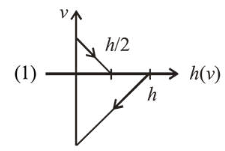2.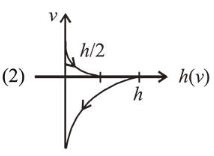3.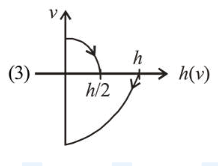4.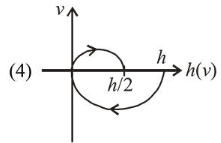Correct Option: , 3

Solution:

(3) For uniformly accelerated/ deaccelerated motion :

$v^{2}=u^{2} \pm 2 g h$

As equation is quadratic, so, $v-h$ graph will be a parabola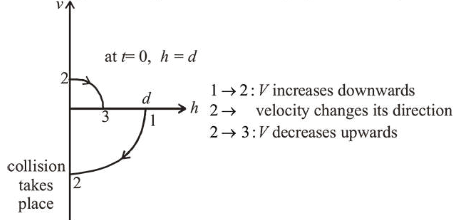Initially velocity is downwards (-ve) and then after collision it reverses its direction with lesser magnitude, i.e. velocity is upwards (+ve).

Note that time $t=0$ corresponds to the point on the graph where $h=d$.

Next time collision takes place at 3 .